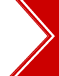Ascent's Courses Business Partnership Jobs @ Ascent Testimonials Success Stories

Functions - Quant/Math - CAT 2013

Question 4 the day: July 16, 2002
The question for the day is from the topic functions.
If f(2, 7) = 57 and f(1, 6) = 37, what is the value of f(3, 10)?
 (1) 107 (2) 127 (3) 147 (4) None of these

Solution:

The function f(a, b) = a3 + b2

f(2, 7) therefore is = 23 + 72 = 8 + 49 = 57 and
f(1, 6) = 13 + 62 = 1 + 36 = 37.

Therefore, f(3, 10) = 33 + 102 = 27 + 100 = 127.

The key to cracking a problem of this kind is the ability with which you can identify the relationship or the function. One suggestion would be to try and see if you can get the pattern in about 30 seconds. If you cannot, do not waste time on the problem. Just skip it and proceed.

 Home | Question Bank | Top B-Schools | US B-Schools | GD-PI | Forums | GRE Preparation Chennai | Doubt Fire © 2002 - 10 ASCENT Education all rights reserved.